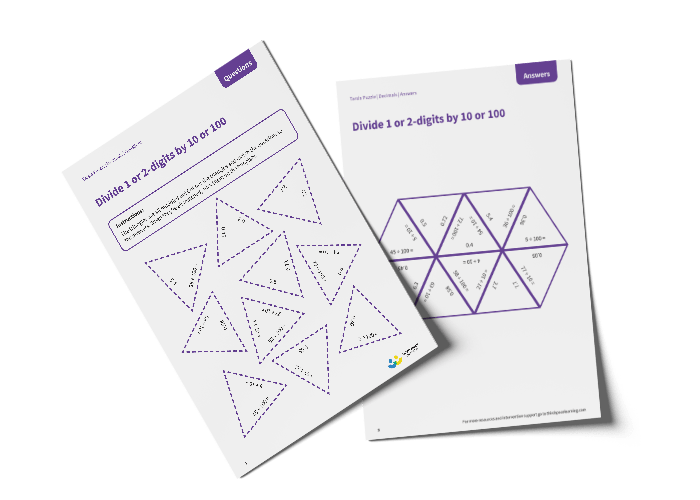# Tarsia Puzzle divide 1 or 2-digits by 10 or 100 (Year 4)

The triangles in the tarsia puzzle have all been mixed up. In this divide 1 or 2-digits by 10 or 100 tarsia puzzle, pupils will need to match the division question to the correct answer to create one large hexagon.

This Year 4 divide 1 or 2-digits by 10 or 100 tarsia puzzle worksheet contains:

• One question sheet
• One template sheet

Year 4

Decimals